Bauer-Fike theorem

As popularized in most texts on computational linear algebra or numerical methods, the Bauer–Fike theorem is a theorem on the perturbation of eigenvalues of a diagonalizable matrix. However, it is actually just one theorem out of a small collection of theorems on the localization of eigenvalues within small regions of the complex plane [a1].

A vector norm is a functional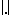on a vectorwhich satisfies three properties:

1)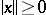, and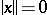if and only if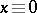.

2)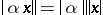for any scalar.

3)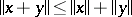(the triangle inequality). A matrix normsatisfies the above three properties plus one more:

4)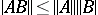(the submultiplicative inequality). An operator norm is a matrix norm derived from a related vector norm:

5). The most common vector norms are: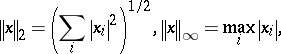where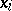denotes theth entry of the vector. The operator norms derived from these are, respectively:where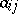denotes theth entry of the matrix. In particular,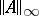is the "maximum absolute row sum" andis the "maximum absolute column sum" . For details, see, e.g., [a2], Sec. 2.2–2.3.

Below, the notationwill denote a vector norm when applied to a vector and an operator matrix norm when applied to a matrix.

Letbe a real or complex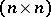-matrix that is diagonalizable (i.e. it has a complete set of eigenvectors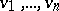corresponding toeigenvalues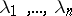, which need not be distinct; cf. also Eigen vector; Eigen value; Complete set). Its eigenvectors make up an-matrix; let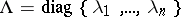be the diagonal matrix. Then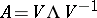. Let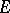be an arbitrary-matrix. The popular Bauer–Fike theorem states that for suchand, every eigenvalueof the matrix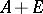satisfies the inequality(a1)

whereis some arbitrary eigenvalue of.

Ifis small, this theorem states that every eigenvalue ofis close to some eigenvalue of, and that the distance between eigenvalues ofand eigenvalues ofvaries linearly with the perturbation. But this is not an asymptotic result valid only as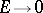; this result holds for any. In this sense, it is a very powerful localization theorem for eigenvalues.

As an illustration, consider the following situation. Letbe an arbitrary-matrix, letbe the diagonal part of, and defineto be the matrix of all off-diagonal entries. Letbe an arbitrary eigenvalue of. The matrix of eigenvectors foris just the identity matrix, so the Bauer–Fike theorem implies that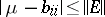, for some diagonal entry. The-norm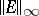is just the maximum absolute row sum over the off-diagonal entries of. In this norm the assertion means that every eigenvalue oflies in a circle centred at a diagonal entry ofwith radius equal to the maximum absolute row sum over the off-diagonal entries. This is a weak form of a Gershgorin-type theorem, which localizes the eigenvalues to circles centred at the diagonal entries, but the radius here is not as tight as in the true Gershgorin theory [a2], sec. 7.2.1, (cf. also Gershgorin theorem).

Another simple consequence of the Bauer–Fike theorem applies to symmetric, Hermitian or normal matrices (cf. also Symmetric matrix; Normal matrix; Hermitian matrix). Ifis symmetric, Hermitian or normal, then the matrixof eigenvectors can be made unitary (orthogonal if real; cf. also Unitary matrix), which means that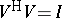, wheredenotes the complex conjugate transpose of. Then, and (a1) reduces to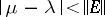. Hence, for symmetric, Hermitian or normal matrices, the change to any eigenvalue is no larger than the norm of the change to the matrix itself.

The Bauer–Fike theorem as stated above has some limitations. The first is that it only applies to diagonalizable matrices. The second is that it says nothing about the correspondence between an eigenvalueof the perturbed matrixand the eigenvalues of the unperturbed matrix. In particular, asincreases from zero, it is difficult to predict how each eigenvalue ofwill move, and there is no way to predict which eigenvalue of the originalcorresponds to any particular eigenvalueof, except in certain special cases such as symmetric or Hermitian matrices.

However, in their original paper [a1], F.L. Bauer and C.T. Fike presented several more general results to relieve the limitation to diagonalizable matrices. The most important of these is the following: Letbe an arbitrary-matrix, and letdenote any eigenvalue of. Then eitheris also an eigenvalue ofor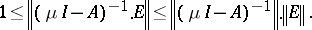(a2)

This result follows from a simple matrix manipulation, using the norm properties given above: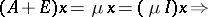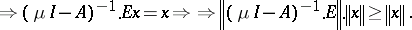Even though this may appear to be a rather technical result, it actually leads to a great many often-used results. For example, ifis diagonalizable, it is easy to show that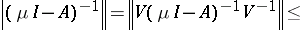which leads immediately to the popular Bauer–Fike theorem (using the fact that the operator norm of a diagonal matrix is just its largest entry in absolute value). One can also repeat the construction, in whichis an arbitrary matrix,is the matrix consisting of the diagonal entries of, and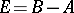is the matrix of off- diagonal entries. Using the norm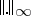, the leftmost inequality in (a2) then reduces to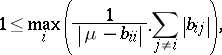leading immediately to the first Gershgorin theorem (see also [a2], Sec. 7.2.1.